# GradientDrawable - When and How to use it in Android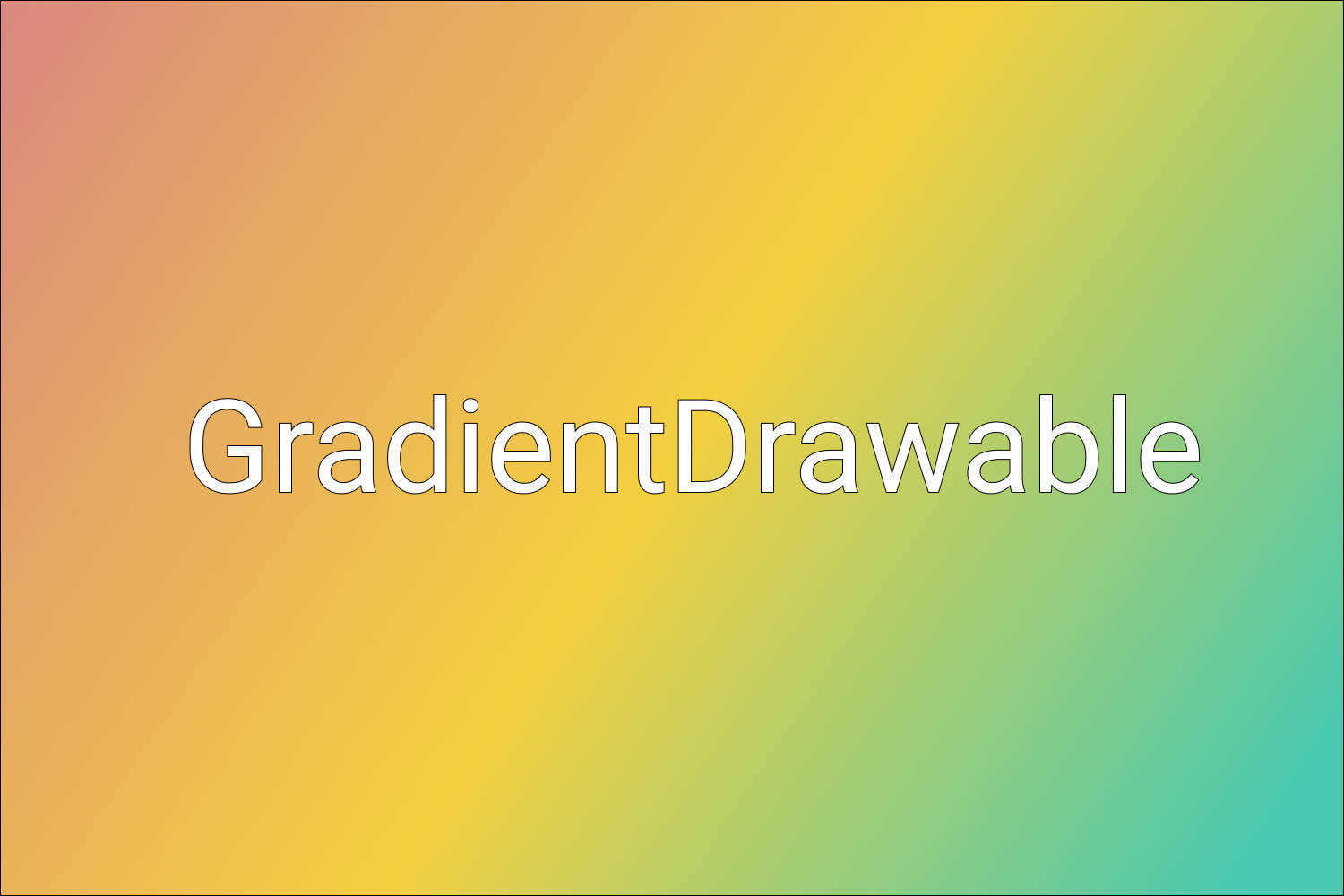The UI of modern-day apps is getting better and better. Designers are trying out different styles and combinations which go best with the Android App. One of the key components of Android being used these days is called GradientDrawable.

A GradientDrawable is drawable with a color gradient for buttons, backgrounds, etc.

Let’s start by taking a basic example of creating a button in Android having an aqua colored background: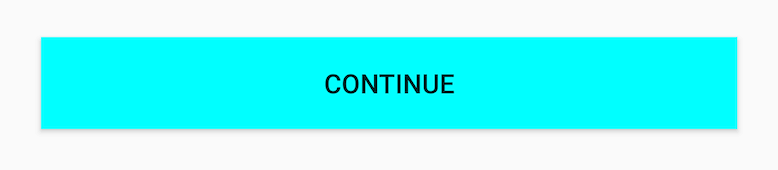This can be done simply by setting the android:background attribute in the XML:

``````<Button
android:layout_width="0dp"
android:layout_height="48dp"
android:layout_marginStart="24dp"
android:layout_marginEnd="24dp"
android:text="Continue"
android:background="#00FFFF"
app:layout_constraintStart_toStartOf="parent"
app:layout_constraintEnd_toEndOf="parent"
app:layout_constraintTop_toTopOf="parent"
app:layout_constraintBottom_toBottomOf="parent" />``````

Here #00FFFF is the color code for Aqua color.

Now, what if you want something like this: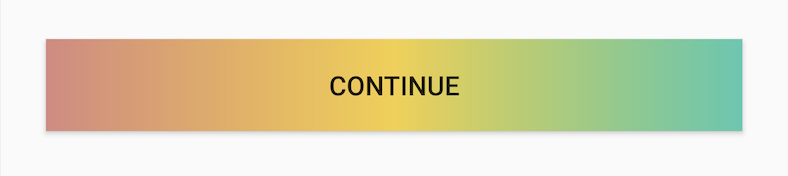For this you need to use gradients.

There are 2 ways to do this:

1. Using a drawable resource in XML

For this, under the drawable package, create a new file tri_color_drawable.xml and enter the following code:

``````<?xml version="1.0" encoding="utf-8"?>
<shape xmlns:android="http://schemas.android.com/apk/res/android" android:shape="rectangle">
android:angle="0"
android:startColor="#D98880"
android:centerColor="#F4D03F"
android:endColor="#48C9B0"/>
</shape>``````

Here we have given a <gradient /> tag and added the three color codes - startColor , centerColor and endColor . We have also given the angle as 0 which denotes LEFT-RIGHT orientation.

Note: Angles can only be multiples of 45. Also, max 3 colors can be specified in XML - startColor, centerColor and endColor. All 3 will occupy equal space.

Then make changes in the <Button /> tag to make it use this background drawable:

``android:background="@drawable/tri_color_drawable"``

Finally, run the app!

We can get the same functionality programmatically as well by using GradientDrawable.

``````val gradientDrawable = GradientDrawable(GradientDrawable.Orientation.LEFT_RIGHT,
intArrayOf(
0XFFD98880.toInt(),
0XFFF4D03F.toInt(),
0XFF48C9B0.toInt()
))``````

Here we have given the orientation as LEFT_RIGHT (which corresponds to 0 we added in XML earlier). We have also given the same three colors we used earlier.

Next, set this gradientDrawable as the background of the button.

``````val continueBtn: Button = findViewById(R.id.continue_btn)

Now run the app!

So why do we need GradientDrawable if the work can be done using XML?

Let’s take a recap of the previous example.Here each color is taking equal space. We can say that color 1 is starting at 0 percent, color 2 at ~33 percent, and color 3 at ~66 percent. What if you don’t want all colors to occupy equal space? Instead, you want something like this: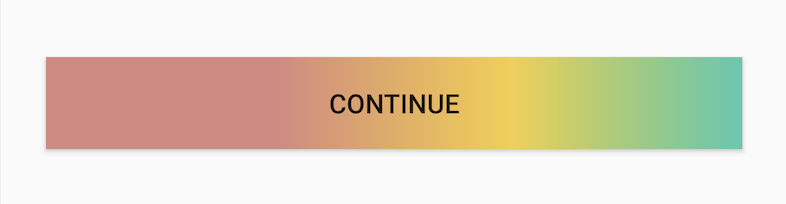If you notice here, color 1 is taking half of the entire space (50 percent) whereas the other two colors are equally covering the remaining space. (25 percent each).

This cannot be achieved via XML. This is where the actual power of GradientDrawable is unleashed.

To achieve the above result, we can do something like this:

``````val gradientDrawable = GradientDrawable(GradientDrawable.Orientation.LEFT_RIGHT,
intArrayOf(
0XFFD98880.toInt(),
0XFFD98880.toInt(),
0XFFF4D03F.toInt(),
0XFF48C9B0.toInt()
))``````

Here color 1 is used twice, hence it will take 50 percent of the total space. Remaining two colors will equally occupy the remaining 50 percent space.

As another example, let’s say you want a 5 color gradient.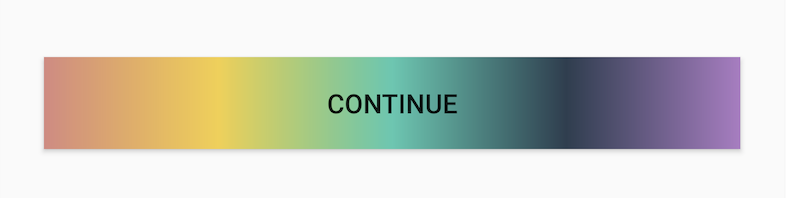This also is not possible via XML but can be easily done using GradientDrawable like this:

``````val gradientDrawable = GradientDrawable(GradientDrawable.Orientation.LEFT_RIGHT,
intArrayOf(
0XFFD98880.toInt(),
0XFFF4D03F.toInt(),
0XFF48C9B0.toInt(),
0XFF2C3E50.toInt(),
0XFFAF7AC5.toInt()
))``````

Here we have given 5 colors and each will cover equal space. You can give as many colors as required.

Lastly, let’s take a closer look at GradientDrawable’s main components:

1. Orientation

It can be one of the orientations as defined in the enum GradientDrawable.Orientation . In our examples, we have used LEFT_RIGHT. Other possible orientations are TOP_BOTTOM , TR_BL , RIGHT_LEFT, etc. They are self-explanatory.

2. Array of Colors

Here we need to provide an array of hexadecimal color values.
For 0XFFD98880,

• 0X -Represents a hexadecimal number.
• FF - Represents the alpha value to be applied to the color. Alpha can be 0 to 100. Here FF represents 100% alpha.
• D98880 - Represents the RRGGBB hexadecimal color value.